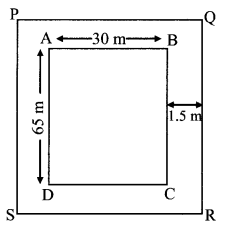# Maharashtra Board Practice Set 45 Class 7 Maths Solutions Chapter 12 Perimeter and Area

Balbharti Maharashtra State Board Class 7 Maths Solutions covers the 7th Std Maths Practice Set 45 Answers Solutions Chapter 12 Perimeter and Area.

## Perimeter and Area Class 7 Practice Set 45 Answers Solutions Chapter 12

Question 1.
If the side of a square is 12 cm, find its area.
Solution:
Area of a square = (side)² = (12)²
= 144 sq. cm.
∴ The area of the square is 144 sq. cm.

Question 2.
If the length of a rectangle is 15 cm and breadth is 5 cm, find its area.
Solution:
Area of a rectangle = length × breadth
= 15 × 5
= 75 sq. cm.
∴ The area of the rectangle is 75 sq. cm.

Question 3.
The area of a rectangle is 102 sq. cm. If its length is 17 cm, what is its perimeter?
Solution:
Area of a rectangle = length × breadth
∴ 102 = 17 × breadth
∴ breadth = $$\frac { 102 }{ 17 }$$ = 6 cm
Perimeter of rectangle = 2 (length + breadth)
= 2 (17 + 6)
= 2 × 23
= 46 cm
∴ The perimeter of rectangle is 46 cm.

Question 4.
If the side of a square is tripled, how many times will its area be as compared to the area of the original square?
Solution:
Let the side of the square be a.
∴ Area of a square = (side)² = a²
New side of the square = 3 × a = 3a
∴ New area of the square = (3a)²
= 9a²
= 9 × area of original square
∴ If the side of a square is tripled, its area will become 9 times the area of the original square.

Maharashtra Board Class 7 Maths Chapter 12 Perimeter and Area Practice Set 45 Intext Questions and Activities

Question 1.
A rectangular playground is 65m long and 30m wide. A pathway of 1.5 m width goes all around the ground, outside it. Find the area of the pathway. (Textbook pg. no. 82)Solution:
The playground is rectangular.
₹ABCD is the playground. Around it is a pathway 1.5 m wide.
Around ₹ABCD we get the rectangle ₹PQRS
Length of new rectangle PQRS = 65 + 1.5 + 1.5 = 68 m
Breadth of new rectangle PQRS = 30 + 1.5 + 1.5 = 33m
Area of path = Area of rectangle PQRS – Area of rectangle ABCD = 68 x 33 – 65 x 30
= 2244 – 1950
= 294 sq m

Question 2.
Is there another way to find the area of the pathway in the problem above? (Textbook pg. no. 82)Solution:
Yes. The area of the pathway can be found by dividing it into rectangles and adding the areas of these rectangles.
Length of rectangle 1 = 30 + 1.5 + 1.5 = 33 m
Breadth of rectangle 1 = 1.5 m
∴ Area of rectangle 1 = 33 x 1.5
= 49.5 sq. m
Area of rectangle 4 = Area of rectangle 1
= 49.5 sq. m.
Length of rectangle 2 = 65 m
breadth of rectangle 2 = 1.5 m
∴ Area of rectangle 2 = 65 x 1.5
= 97.5 sq. m.
Area of rectangle 3 = area of rectangle 2
= 97.5 sq. m.
∴ Area of pathway = Sum of area of the 4 rectangles = 49.5 + 49.5 + 97.5 + 97.5
= 294 sq. m.

Question 3.
The length and the width of a mobile phone are 13 cm and 7 cm respectively. It has a screen PQRS as shown in the figure. What is the area of the screen? (Textbook pg. no. 82)Solution:
ABCD is the rectangle formed by the edges of the mobile. PQRS is the rectangle formed by leaving a 1.5 cm wide edge alongside AB, BC, and DC, and a 2 cm edge alongside DA.
Length of rectangle PQRS = 9.5 cm
Breadth of rectangle PQRS = 4 cm
Area of screen = Area of rectangle PQRS = 9.5 x 4
= 38 sq .cm

Scroll to Top